#### You may also like### Some(?) of the Parts

A circle touches the lines OA, OB and AB where OA and OB are perpendicular. Show that the diameter of the circle is equal to the perimeter of the triangleA 1 metre cube has one face on the ground and one face against a wall. A 4 metre ladder leans against the wall and just touches the cube. How high is the top of the ladder above the ground?### At a Glance

The area of a regular pentagon looks about twice as a big as the pentangle star drawn within it. Is it?

# Far Horizon

##### Age 14 to 16Challenge Level

The solution below is based on the one sent in by Barinder of Langley Grammar School. We had quite a number of correct, well laid out solutions to this problem this month including those from Roy of Allerton High School, Dan (no address given) and Calum of Wayland High School.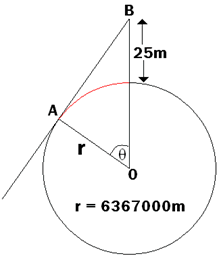Although definitely not in proportion, this makes the problem seem a lot easier. The question is asking for the length of the arc I have coloured red. To get this, I decided to find the angle $\theta$ on the diagram, and use the equation

Arc Length $= \frac {\theta}{360} \times 2 \pi r$ where $\theta$ is measured in degrees and r is the radius.

$\angle OAB = 90^\circ$, since it is where a tangent and a radius of a circle meet - it is a circle theorem.

Thus, the triangle AOB can be drawn as follows: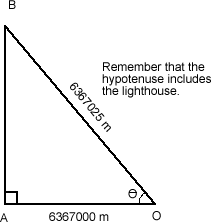We can now use trigonometry to find $\theta$:

\begin{align*} \cos \theta &= \frac {6367000}{6367025} \\ \cos \theta &= 0.99999607 \\ \theta &= \cos^{-1} (0.99999607) = 0.1606^\circ \mbox{(4 d.p.)}\end{align*}

Substitute this into the equation for the arc length of a circle earlier to obtain the length required:

Arc Length $= \frac {0.1606}{360} \times 2\pi r.$

Arc Length $= 0.000446 \times 2 \times \pi \times 6367000 = 17,842.3m = 17.8 km$

The cliffs of Dover
For this next part, we are given the arc length, since this corresponds to the distance between England and France. The diagram is therefore: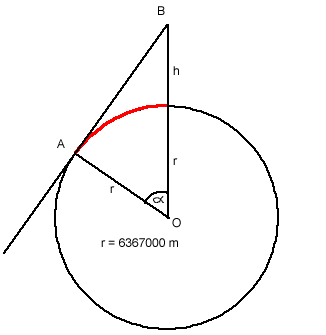This is essentially the reverse of the previous question. We need to find the angle $\alpha$ first, and to do this, we consider the arc length of the sector OAD of the circle:

Arc Length $= \frac {\alpha}{360} \times 2 \pi r$.

So $32000 = \frac {\alpha}{360} \times 2 \times \pi \times 6367000$.

Then $32000 \times 360 = \alpha \times 2 \times \pi \times 6367000$.

So $\alpha = 0.288^\circ \mbox{(3 d.p.)}$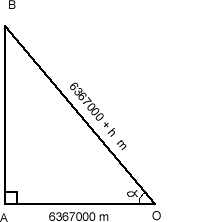Since we now have the angle $\alpha$, we can consider the triangle AOB:

$\cos \alpha = \frac {6367000}{6367000 + h}$.

So $6367000 + h = \frac {6367000}{\cos (0.288)}$.

So $6367000 + h = 6367080.415$

$h = 6367080.415 - 6367000 = 80.415$ m high.

Thus, the cliffs of Dover are $80.4$ metres high.

Clare's distance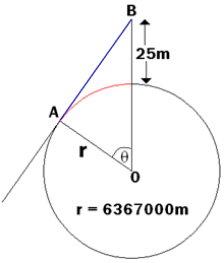In this case, Clare has found the distance AB, shown in blue on this copy of the original diagram.

Using Pythagoras' Theorem,
$AB^2+6367000^2=6367025^2$
$\Rightarrow AB^2+40538689000000=40539007350625$
$\Rightarrow AB^2=318350625$
$\Rightarrow AB=17842.3828...\approx17842.4$ metres

This is very similar to the red distance, which we found to be $17842.3$ metres - they only differ by about $5$ cm!

To explain why these distances are so similar, a more representative diagram is helpful. Really, the lighthouse is very small compared to the Earth, and we found that $\theta=0.1606^\circ$, so the triangle should be very skinny!

In the diagram below, the lighthouse is still too big relative to the Earth as the angle is still several degrees (rather than $0.1606^\circ$), but you can see that the blue and red distances must be similar.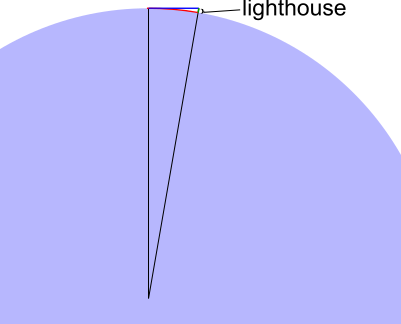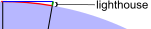What happens as the lighthouse gets even smaller reltive to the Earth - or as $\theta$ gets even closer to $0$?